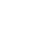# 30 Dollars An Hour – How Much A Year? (Example Budget Included)

## How Much Is 30 Dollars An Hour Per Year?

To calculate how much you will get paid per year, let’s assume you work 52 weeks of the year (with 2 weeks paid time off). If you are working a full-time job, you will be working 40 hours per week on average.

40 hours multiplied by 52 weeks is 2,080 working hours in a year.

\$30 per hour multiplied by 2,080 working hours per year is an annual income of \$62,400 per year.

## What If You Don’t Get Paid Time Off?

If you don’t get paid vacation, let’s assuming you are working 50 weeks of the year (with 2 weeks unpaid time off). And we’ll assume you work an average of 40 hours per week.

40 hours multiplied by 50 weeks is 2,000 working hours in a year.

Now simply multiply your hourly rate by the number of working hours in the year.

\$30 per hour multiplied by 2,000 working hours per year is an annual income of \$60,000 per year.

## How Many Working Days Are In A Year (2020)?

For a more accurate calculation, you can calculate EXACTLY how many working days are in the year.

For the year 2020, it’s a leap year, so there are 366 days in the year. Here’s how the days break down:

• 262 Weekdays
• 104 Weekend Days (woohoo!)

Assuming your are working each weekday, 8 hours per day, here is how many hours you will work:

262 work days multiplied by 8 hours per day is 2,096 working hours in 2020

\$30 an hour multiplied by 2,096 working hours is \$62,880 income per year

*This does not include any overtime hours worked

## How Much Is \$30 An Hour Per Month?

If you want to see how much \$30 an hour is a month, we need to know how many working hours there are in a month.

If we divide the total working hours in a year by 12 (months), we can see how many working hours are in a month.

2,096 hours a year divided by 12 is about 175 working hours per month on average.

So to calculate your monthly income, see below:

\$30 an hour multiplied by 175 hours per month is \$5,250 per month income on average.

## How Much Is \$30 An Hour Per Week?

If you want to break it out by week, let’s assume your working a normal 40-hour week.

So to calculate your weekly income, see below:

\$30 an hour multiplied by 40 hours per week is \$1,200 per week income.

## How Much Is \$30 An Hour Per Day?

Let’s see how much you can make per day on \$30 an hour. Assuming you are working a typical 8-hour workday, here’s the answer:

\$30 an hour multiplied by 8 hours per day is \$240 per day income.

## Example Budget For \$30 Per Hour

Now that you know how much you can make per year, per month and weekly, let’s see how a typical budget can look making \$30 per hour.

Remember, for your budget, you need to calculate the estimated take-home pay.

Taking the \$30 an hour monthly income of \$5,250, minus taxes, the estimated take home pay is \$4,043 (varies by state and paycheck deductions)

Sample Monthly Budget For \$30 An Hour:

As you can see, this budget allows for saving a decent amount (\$500 per month), and would be great for a young couple, possibly renting an apartment. I recommend putting the \$500 per month into your work 401k account or a Roth IRA for best results.

If looking at buying a house on \$30 an hour, make sure you can put 20% down and keep monthly housing costs under \$1,100 per month.

Don’t like the budget? Grab your own budget template below and plug in your numbers for your \$30 an hour budget.

Jacob Wade has been a nationally-recognized personal finance expert for the past decade. He has written professionally for The Balance, The Spruce, LendingTree, Investing Answers, and other widely-followed sites.
He’s also been a featured expert on CBS News, MSN Money, Forbes, Nasdaq, Yahoo! Finance, Go Banking Rates, and AOL Finance.

In 2018, Jacob quit his job and his family decided to sell everything (including their home) to take off on an adventure. They traveled the country in an RV for nearly 3 years, visiting over 38 states, 20+ national parks and eventually settling in the sunshine state!

### 3 thoughts on “30 Dollars An Hour – How Much A Year? (Example Budget Included)”

1.Wait? where in America can you live off of \$1011/month??

•2.